Chapter 5 Class 12 Continuity and Differentiability

Class 12
Important Questions for exams Class 12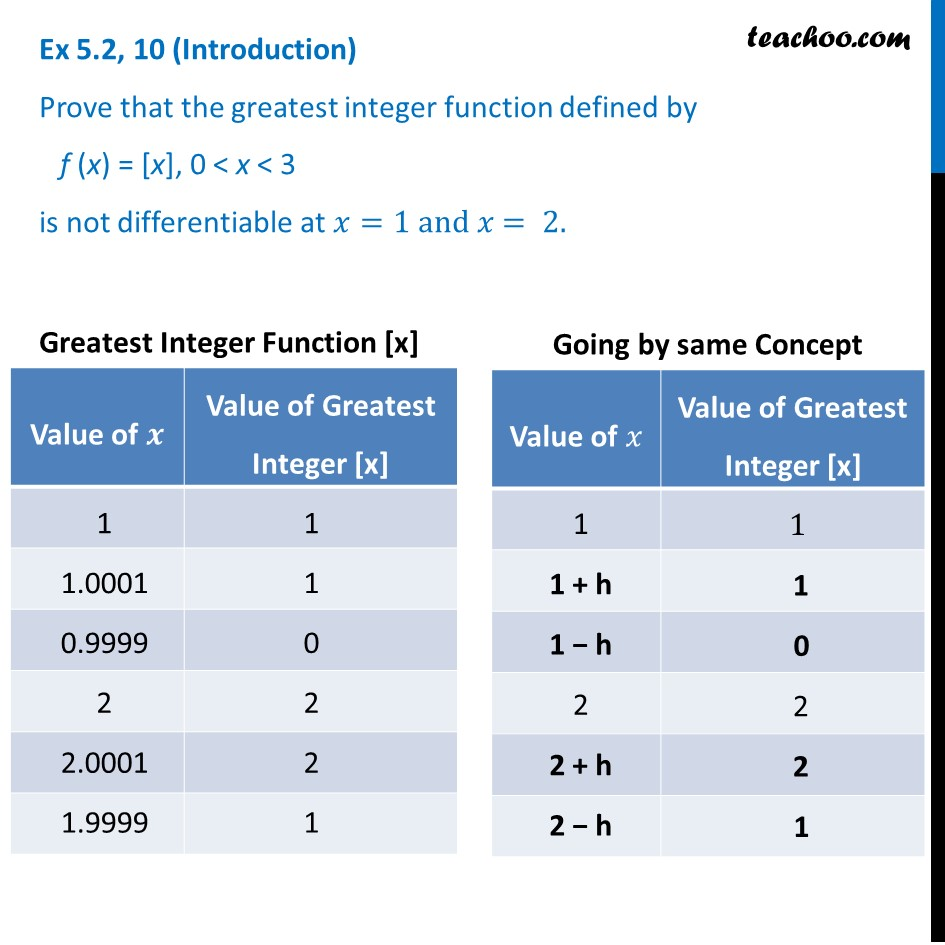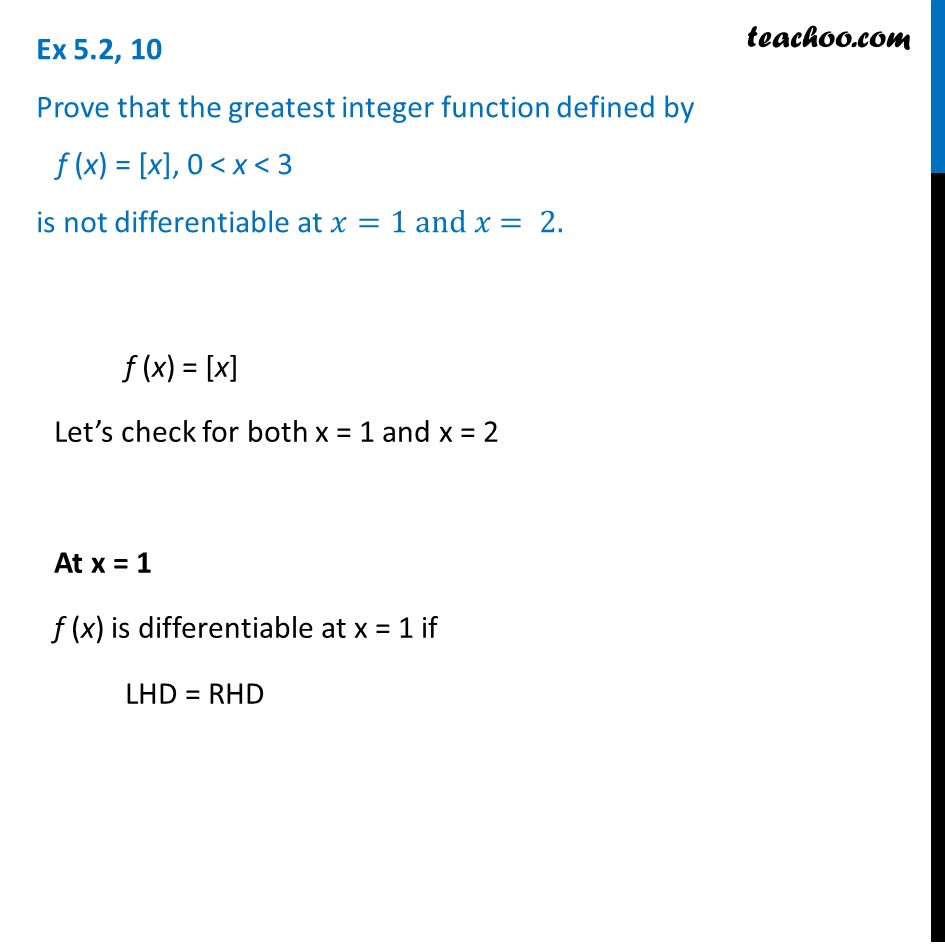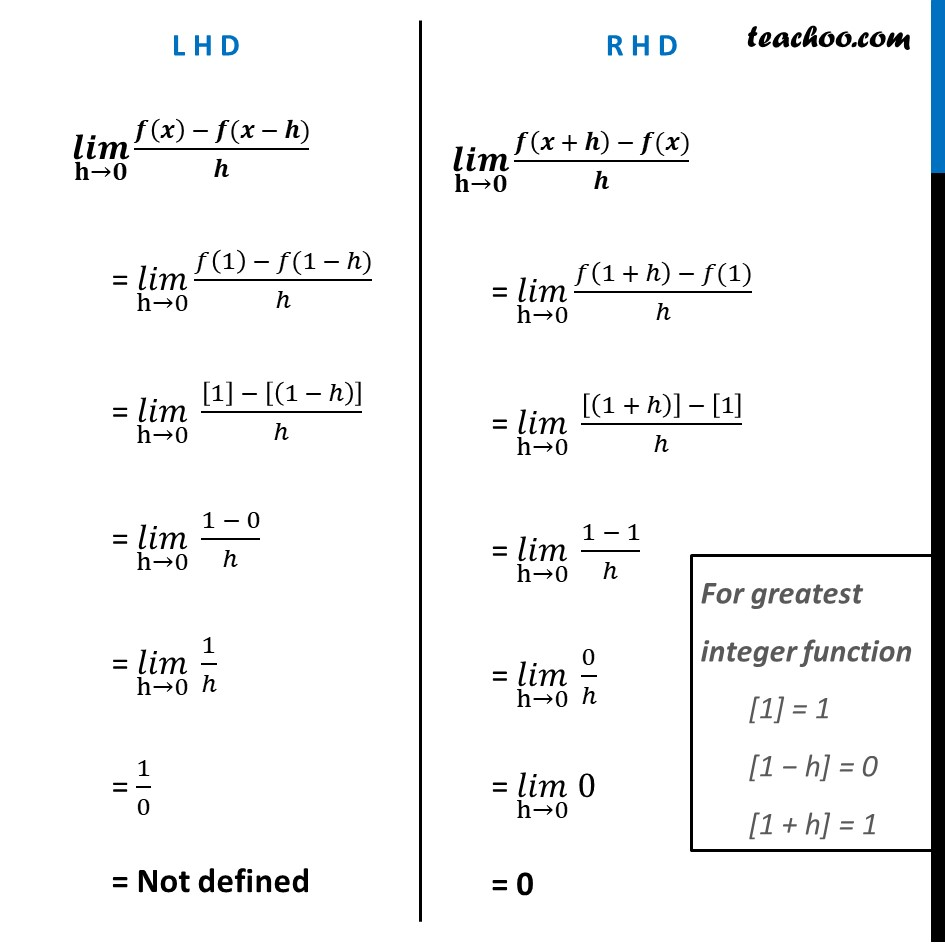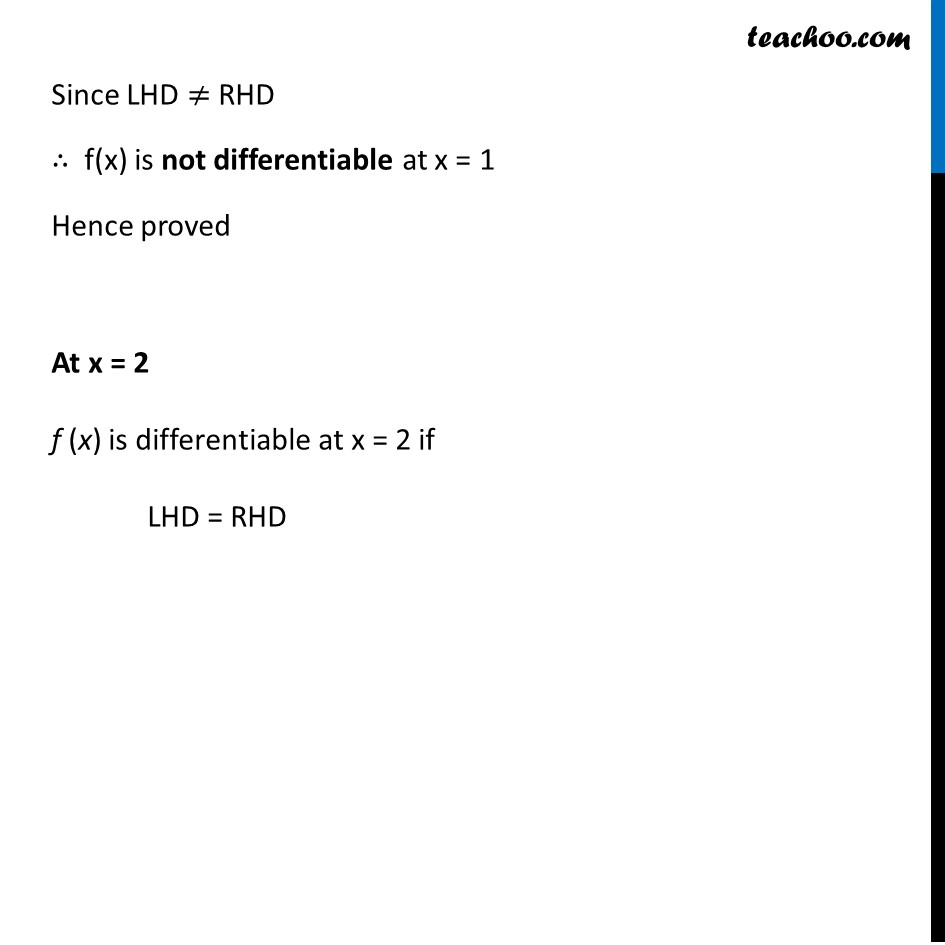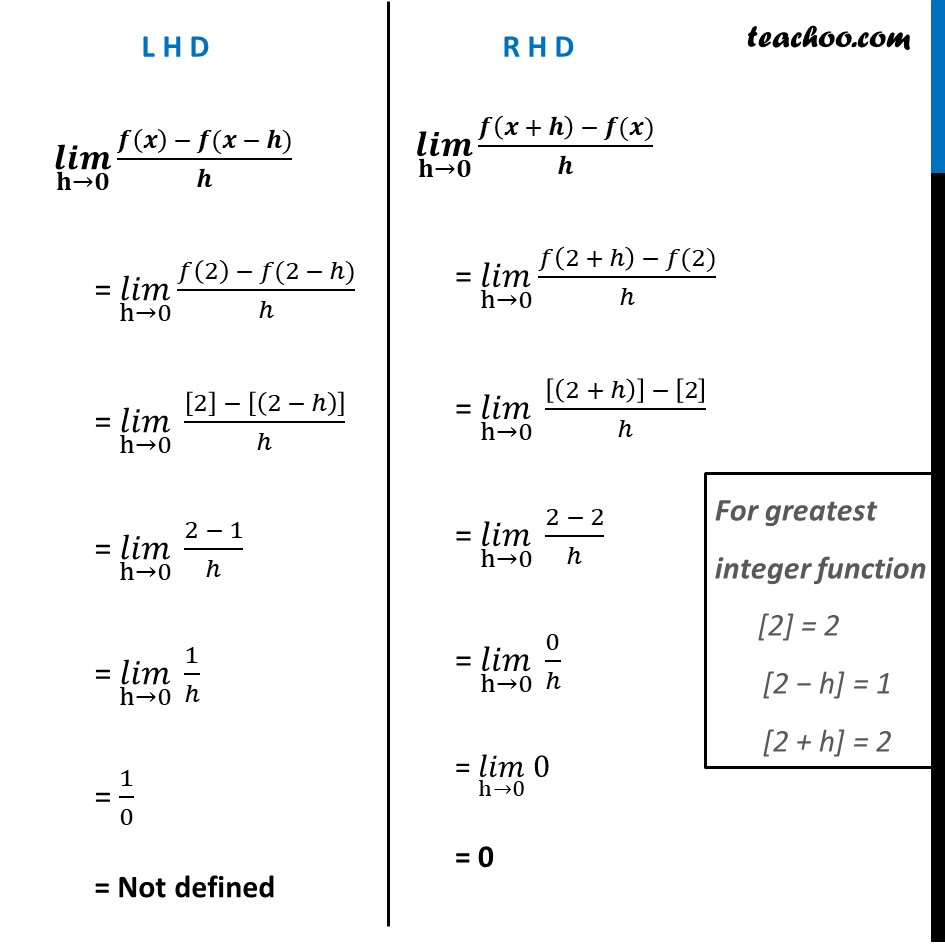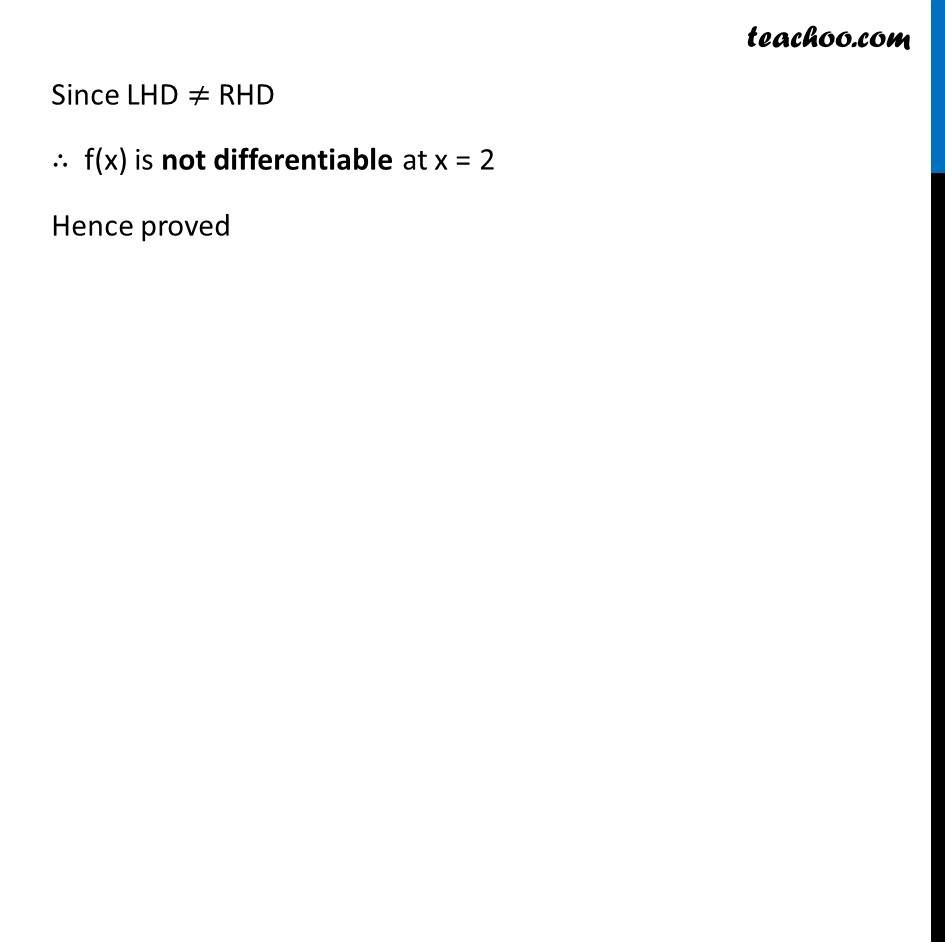Learn in your speed, with individual attention - Teachoo Maths 1-on-1 Class

### Transcript

Ex 5.2, 10 (Introduction) Prove that the greatest integer function defined by f (x) = [x], 0 < x < 3 is not differentiable at 𝑥=1 and 𝑥= 2. Ex 5.2, 10 Prove that the greatest integer function defined by f (x) = [x], 0 < x < 3 is not differentiable at 𝑥=1 and 𝑥= 2. f (x) = [x] Let’s check for both x = 1 and x = 2 At x = 1 f (x) is differentiable at x = 1 if LHD = RHD (𝒍𝒊𝒎)┬(𝐡→𝟎) (𝒇(𝒙) − 𝒇(𝒙 − 𝒉))/𝒉 = (𝑙𝑖𝑚)┬(h→0) (𝑓(1) − 𝑓(1 − ℎ))/ℎ = (𝑙𝑖𝑚)┬(h→0) ( − [(1 − ℎ)])/ℎ = (𝑙𝑖𝑚)┬(h→0) (1 − 0)/ℎ = (𝑙𝑖𝑚)┬(h→0) 1/ℎ = 1/0 = Not defined (𝒍𝒊𝒎)┬(𝐡→𝟎) (𝒇(𝒙 + 𝒉) − 𝒇(𝒙))/𝒉 = (𝑙𝑖𝑚)┬(h→0) (𝑓(1 + ℎ) − 𝑓(1))/ℎ = (𝑙𝑖𝑚)┬(h→0) ([(1 + ℎ)] − )/ℎ = (𝑙𝑖𝑚)┬(h→0) (1 − 1)/ℎ = (𝑙𝑖𝑚)┬(h→0) 0/ℎ = (𝑙𝑖𝑚)┬(h→0) 0 = 0 For greatest integer function  = 1 [1 − h] = 0 [1 + h] = 1 Since LHD ≠ RHD ∴ f(x) is not differentiable at x = 1 Hence proved At x = 2 f (x) is differentiable at x = 2 if LHD = RHD L H D (𝒍𝒊𝒎)┬(𝐡→𝟎) (𝒇(𝒙) − 𝒇(𝒙 − 𝒉))/𝒉 = (𝑙𝑖𝑚)┬(h→0) (𝑓(2) − 𝑓(2 − ℎ))/ℎ = (𝑙𝑖𝑚)┬(h→0) ( − [(2 − ℎ)])/ℎ = (𝑙𝑖𝑚)┬(h→0) (2 − 1)/ℎ = (𝑙𝑖𝑚)┬(h→0) 1/ℎ = 1/0 = Not defined R H D (𝒍𝒊𝒎)┬(𝐡→𝟎) (𝒇(𝒙 + 𝒉) − 𝒇(𝒙))/𝒉 = (𝑙𝑖𝑚)┬(h→0) (𝑓(2 + ℎ) − 𝑓(2))/ℎ = (𝑙𝑖𝑚)┬(h→0) ([(2 + ℎ)] − )/ℎ = (𝑙𝑖𝑚)┬(h→0) (2 − 2)/ℎ = (𝑙𝑖𝑚)┬(h→0) 0/ℎ = (𝑙𝑖𝑚)┬(h→0) 0 = 0 For greatest integer function  = 2 [2 − h] = 1 [2 + h] = 2 Since LHD ≠ RHD ∴ f(x) is not differentiable at x = 2 Hence proved